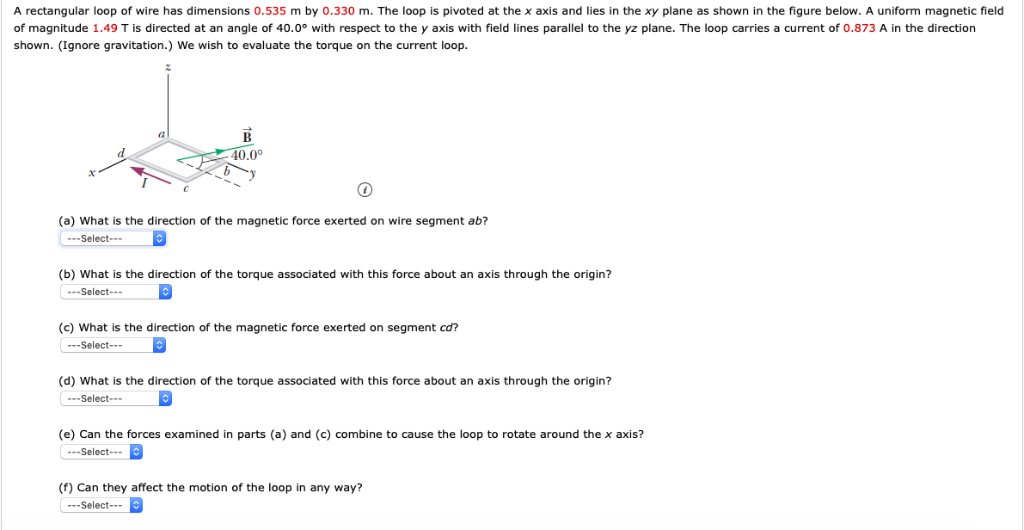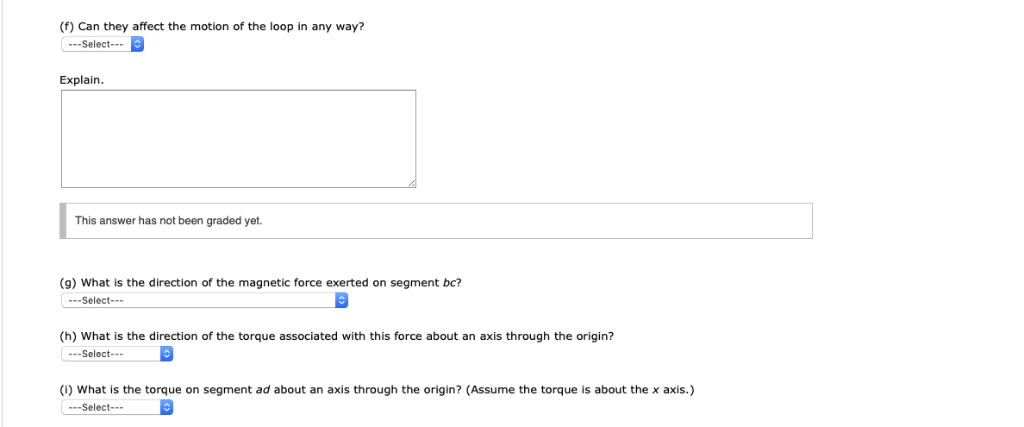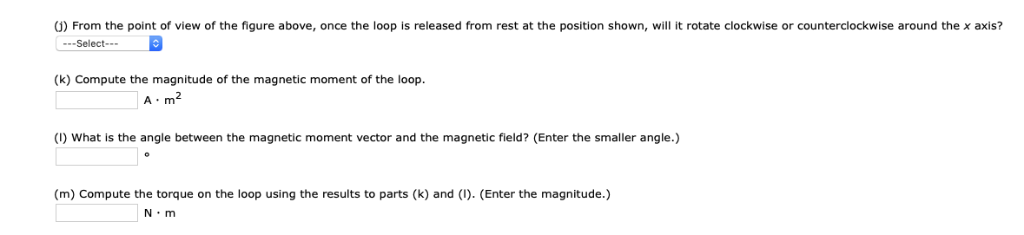# Question A rectangular loop of wire has dimensions 0.535 m by 0.330 m. The loop is pivoted at the x axis and lies in the xy plane as shown in the figure below. A uniform magnetic field of magnitude 1.49 T is directed at an angle of 40.0 with respect to the y axis with field lines parallel to the yz plane. The loop carries a current of 0.873 A in the direction shown. (Ignore gravitation.) We wish to evaluate the torque on the current loop. 40.0 (a) What is the direction of the magnetic force exerted on wire segment ab? Select-- (b) What is the direction of the torque associated with this force about an axis through the origin? Select. (c) What is the direction of the magnetic force exerted on segment cd? --Select- (d) What is the direction of the torque associated with this force about an axis through the origin? -Select (e) Can the forces examined in parts (a) and (c) combine to cause the loop to rotate around the x axis? Select (f) Can they affect the motion of the loop in any way? --Select (f) Can they affect the motion of the loop in any way? --Select Explain This answer has not been graded yet. (9) What is the direction of the magnetic force exerted on segment bc? -Select- (h) What is the direction of the torque associated with this force about an axis through the origin? Select (i) What is the torque on segment ad about an axis through the origin? (Assume the torque is about the x axis.) -Select- 0) From the point of view of the figure above, once the loop is released from rest at the position shown, will it rotate clockwise or counterclockwise around the x axis? (k) Compute the magnitude of the magnetic moment of the loop. A m2 (I) What is the angle between the magnetic moment vector and the magnetic field? (Enter the smaller angle.) (m) Compute the torque on the loop using the results to parts (k) and (I). (Enter the magnitude.) N m